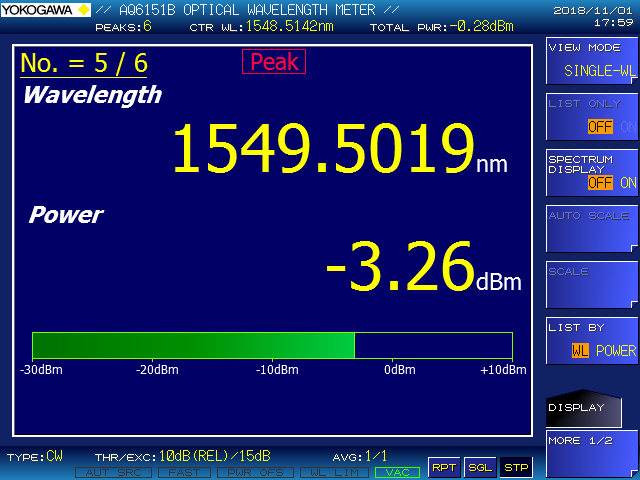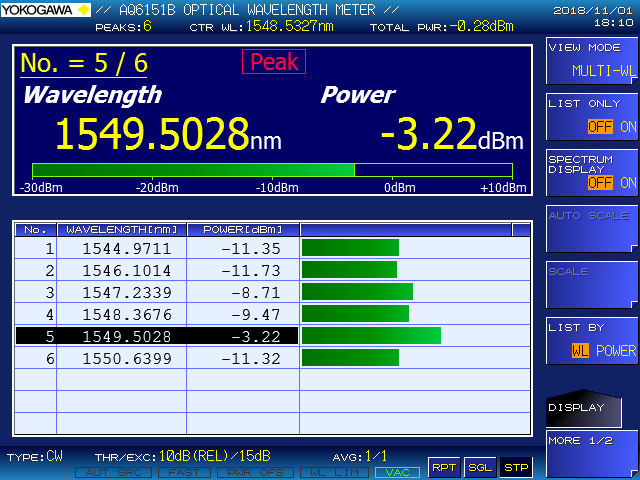Optical Wavelength Meter

A Michelson interferometer-based optical wavelength meter with high measurement performance and excellent cost performance that meets measurement needs from optical devices to optical transmission equipment.

There are 12 types depending on the combination of wavelength accuracy, wavelength range, and number of detection wavelengths.
Wavelength accuracy (Standard / High accuracy)
Standard: ±0.7ppm (AQ6150B), High accuracy: ±0.2ppm (AQ6151B)
Wavelength range (Standard / Extended / Wide-range)
Standard: Supports optical communication wavelengths of O to L bands
Expansion: Supports wavelengths of CWDM transceivers as wll as the O to L bands
Wide-range: Supports wavelengths of pump lasers as wll as the extended O to L bands
Number of wavelengths (Single wavelength / Multi-wavelength)
Multi-wavelength: simultaneously measures multiple wavelengths such as WDM signals
Single wavelength: measures only a single wavelength such as an output from a wafer or an LD chip

AQ6150B Optical Wavelength Meter

The AQ6150B measures the wavelength and power levels of both CW and Modulated optical signals from transceivers and WDM transmission systems.

• Wavelength range: 900 to 1700 nm
• Wavelength accuracy: ±0.7 ppm

AQ6151B Optical Wavelength Meter

The AQ6151B accurately measures the wavelength and power level of both CW and modulated optical signals from tunable lasers and DWDM transmission systems.

• Wavelength range: 900 to 1700 nm
• Wavelength accuracy: ±0.2 ppm

Optical wavelength meter AQ6150B

 Single Wavelength Meter AQ6150B-10-SW Single Wavelength Meter AQ6150B-20-SW Single Wavelength Meter AQ6150B-30-SW O to L-band（Standard） O to L-band（Extended） Wide-range Wavelength range: 1270 to 1650 nm Wavelength accuracy: ±0.7 ppm Number of wavelength:1 Min.measurement time: 0.2 s or less Wavelength range: 1200 to 1700 nm Wavelength accuracy: ±0.7 ppm Number of wavelength:1 Min. measurement time: 0.2 s or less Wavelength range: 900 to 1700 nm Wavelength accuracy: ±0.7 ppm Number of wavelength:1 Min. measurement time: 0.2 s or less

 Multi Wavelength Meter AQ6150B-10-MW Multi Wavelength Meter AQ6150B-20-MW Multi Wavelength Meter AQ6150B-30-MW O to L-band（Standard） O to L-band（Extended） Wide-range Wavelength range: 1270 to 1650 nm Wavelength accuracy: ±0.7 ppm Number of wavelengths:1024 (max.) Min.measurement time: 0.2 s or less Wavelength range: 1200 to 1700 nm Wavelength accuracy: ±0.7 ppm Number of wavelengths:1024 (max.) Min. measurement time: 0.2 s or less Wavelength range: 900 to 1700 nm Wavelength accuracy: ±0.7 ppm Number of wavelengths:1024 (max.) Min. measurement time: 0.2 s or less

High accuracy optical wavelength meter AQ6151B

 Single Wavelength Meter AQ6151B-10-SW Single Wavelength Meter AQ6151B-20-SW Single Wavelength Meter AQ6151B-30-SW O to L-band（Standard） O to L-band（Extended） Wide-range Wavelength range: 1270 to 1650 nm Wavelength accuracy: ±0.2 ppm Number of wavelength:1 Min.measurement time: 0.2 s or less Wavelength range: 1200 to 1700 nm Wavelength accuracy: ±0.2 ppm Number of wavelength:1 Min. measurement time: 0.2 s or less Wavelength range: 900 to 1700 nm Wavelength accuracy: ±0.2 ppm Number of wavelength:1 Min. measurement time: 0.2 s or less

 Multi Wavelength Meter AQ6151B-10-MW Multi Wavelength Meter AQ6151B-20-MW Multi Wavelength Meter AQ6151B-30-MW O to L-band（Standard） O to L-band（Extended） Wide-range Wavelength range: 1270 to 1650 nm Wavelength accuracy: ±0.2 ppm Number of wavelengths:1024 (max.) Min.measurement time: 0.2 s or less Wavelength range: 1200 to 1700 nm Wavelength accuracy: ±0.2 ppm Number of wavelengths:1024 (max.) Min. measurement time: 0.2 s or less Wavelength range: 900 to 1700 nm Wavelength accuracy: ±0.2 ppm Number of wavelengths:1024 (max.) Min. measurement time: 0.2 s or less

Display exampleSingle Wavelength MeterMulti Wavelength Meter

Overview:

This paper describes a compact optical channel monitor and a delayed interferometer having free-space optical elements such as lenses or mirrors, as an application of microoptics. These devices have been developed to be built into dense wavelength division multiplexing (DWDM) transmission systems. These optics use a Gaussian beam which is emitted through single-mode optical fibers and located near the optical axis. This paper explains the optical designs of these devices based on the Gaussian beam's behavior.
SANPEI Yoshihiro*1  SUZUKI Yasuyuki*2   IEMURA Kouki*3   ASANO Junichirou*3

*1